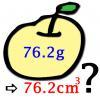#### You may also like### Far Horizon

An observer is on top of a lighthouse. How far from the foot of the lighthouse is the horizon that the observer can see?### Data Matching

Use your skill and judgement to match the sets of random data.### More or Less?

Are these estimates of physical quantities accurate?

# Big and Small Numbers in the Physical World

##### Age 14 to 16Challenge Level

1. Let's change miles per hour to meters per second:  1 mile = 1.61 km and 1 hour = 3600 seconds. Thus $c=3\times 10^8$m/s = $3\times 10^8$$\times 3600/1610 mph =6.7\times 10^8mph. The fastest car in 2010 was the Bugatti Veyron with max speed 250 mph. The speed of light is 6.7\times 10^8 / 250 = 2.7\times 10^6 times bigger than the speed of a sport car. 2. We are given that a diameter of the galaxy is D = 100,000 ly and a thickness about d = 1000 ly. We can approximate our galaxy as a cylinder then calculate a volume of the galaxy. V = d\times \pi (D/2)^2. An average number of starts in the galaxy is N = 250 billion stars. Now, we can find out a density of the stars: N/V = (250\times 10^9) : (1000\times \pi (100,000/2)^2) = 0.032 stars/ly^3. Thus, one star occupies the volume which is equal 1/0.032 ly^3=31$$ly^3$. In order to find the average distance between stars we take a cube root of 31 to get that the distance is about 3.2 light years.

3. Mass of lead $m = 1 t = 1000 kg = 1\times 10^6 g$. Density of lead $11.34$g/cm$^3$. Thus, the volume of 1 tonne of lead is $V =(1\times 10^6) /(11.34)$ cm$^3$ = 88180 cm$^3$.

We can imagine this volume as a cube with side length 44.5 cm or as a ball with diameter $55.2 cm$, for a comparison the diameter of football ball is 14-16 cm.

4. Firstly, lets find a mass of a roll of aluminum kitchen foil. A thickness of foil is about 20 micrometes, lenght is 10 meters and width is about 370 milimetres. Hence, the volume of a roll $V = 20\times 10^{-6} \times 10\times 370\times 10^{-3} m^3= 7.4\times 10^{-5} m^3$. The density of aluminum is $7874 kg/m^3$. The mass of this roll is $7.4\times 10^-5\times 7874 = 0.58 kg$. It takes around 2 kg of bauxite (aluminum ore) to make  1 kg of pure aluminum metal.   Thus, we need about 1.2 kg of aluminum ore.

5. One AA zinc-carbon battery has a capacity of 1100 mAh.  For example one AA Alkaline battery has much bigger capacity of 2700 mAh. A power being used by a laptop is about 60 watts and AC adapter changes voltage from 240 volts to average 20 volts. The formula which relates power with the voltage and the current is $P = I\times U$ where P is power, I the current and U is the voltage. The electric current is a flow of electric charge. Thus, the charge required is $60/20\times 1 = 3$Ah. We can conclude that we need about three AA zinc-carbon batteries or one good Alkaline battery to run a laptop for one hour.

6. A mass of one staple is about 30 mg. A staple is made of stainless steel which consists mostly of iron. The molar mass of iron is $M = 55.85 g/mol$ and  Avogadro's number is $6.02\times 10^23$ 1/mol. Hence, the number of atoms in a staple is $N = 6.02\times 10^{23}\times 0.03/55.85 = 3.2\times 10^{20}$

7. Energy stored in a staple is $E = mc^2$ where mass of a stample is $m = 30$ mg and  the speed of light is $c = 3\times 10^8$ m/s. So, $E = 2.7\times 10^{15}$ J. Power is the rate at which energy is transferred. If we assume that a laptop use 60 W power then it could run for $2.7\times 10^{15} : 60$ s= $4.5\times 10^{13}$ s = 1.4 million years.

8. Suppose a volume of room (class) is $10\times 6\times 3 m^3= 180 m^3$. The specific heat capacity of air when a pressure is constant $c = 1012$ $J/(kg\times K)$. The energy required to raise the temperature by T = 1$^\circ$C could be calculated by $E = mcT$ where m is the mass of air in the room. A density of air is $1.2 kg/m^3$). Thus, $E = 1.2\times 180\times 1012\times 1 = 2.2\times 10^5$ J. The heat of combustion is the energy released when a compound undergoes complete combustion. For the natural gas it is $45 MJ/kg$.  The mass of natural gas required to raise the temperature by T = 1$^\circ$C is $2.2\times 10^5 : 45\times 10^6$ = 5 grams. The density of natural gas is about $0.8 kg/m^3$. Thus, we need $V = 6.25\times 10^{-3} m^3$ of natural gas. Most heating isn't perfect however, and supposing it is 50% efficient we actually need twice as much gas! That's $1.25\times10^{-2} m^3$. In Britain gas is billed according to energy, not by volume, and is priced per kilowatt-hour ($kWh$). A typical price could be £$0.038$ per $kWh$. On average we expect gas to have a calorific value of $40 MJ/m^3$, which means that one metre cubed of gas produces $40 MJ$ of energy. Thus our $1.25\times10^{-2} m^3$ of gas holds roughly $0.5 MJ$ of energy. Convert this to $kWh$ using the factor $1 kWh = 3.6 MJ$ to get that we need $0.139 kWh$ of gas. Using the price above we find that this costs merely half a penny!